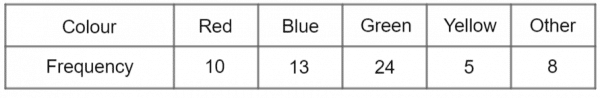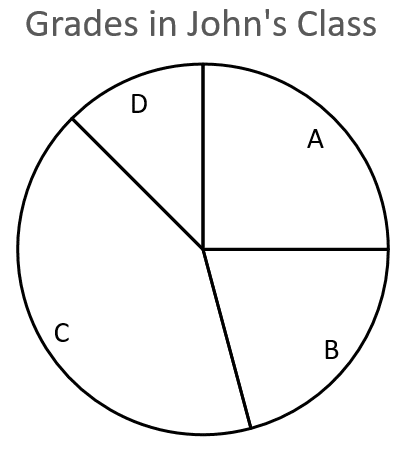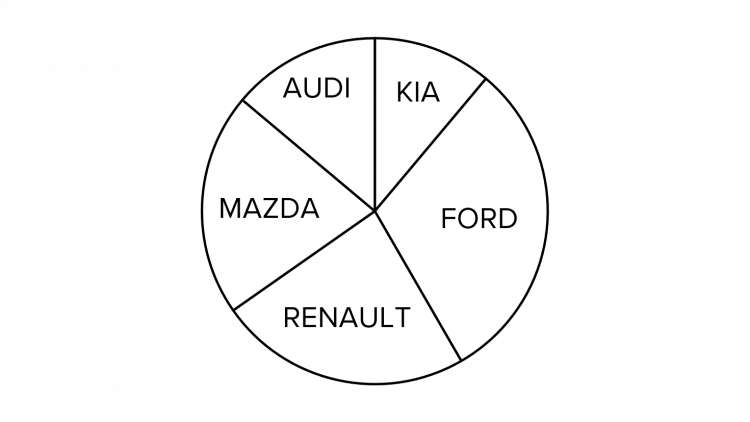# Pie Charts

GCSEKS3Level 4-5AQACambridge iGCSEEdexcelEdexcel iGCSEOCRWJEC

## Pie Charts

A pie chart is a circular chart used to compare the sizes of data sets in relation to one another.

Make sure you are happy with the following topics before continuing.

Level 4-5GCSEKS3AQAEdexcelOCRWJECCambridge iGCSEEdexcel iGCSE

## How to Draw a Pie Chart

Start by drawing a circle with a compass, and then measure the angles with a protractor to make sure we get them right.

To measure the size of each slice, we use the size of the angle that it will take up, out of the total $360\degree$ of the circle. To calculate the angle, we use the following formula:

$\textcolor{black}{\text{angle }}=\dfrac{\textcolor{black}{\text{number of things in that category}}}{\textcolor{black}{\text{total number of things}}}\times \textcolor{black}{360\degree}$

Note: Knowing how to use a compass and protractor will come in handy for other topics and will save time in the exam so make sure you get plenty of practise.

Level 4-5GCSEKS3AQAEdexcelOCRWJECCambridge iGCSEEdexcel iGCSE
Level 4-5GCSEKS3AQAEdexcelOCRWJECCambridge iGCSEEdexcel iGCSE

## Example 1: Drawing a Pie Chart

Brian asks $60$ people what their favourite colour is and separates the answers into $5$ categories. His results are shown in the table below. Draw a pie chart to display Brian’s results.

[4 marks]To draw the pie chart, we need to calculate the size of the angle needed for each category using the formula.$\text{‘Red’ angle }=\dfrac{10}{60}\times 360=60\degree$

$\text{‘Blue’ angle }=\dfrac{13}{60}\times 360=78\degree$

$\text{‘Green’ angle }=\dfrac{24}{60}\times 360=144\degree$

$\text{‘Yellow’ angle }=\dfrac{5}{60}\times 360=30\degree$

$\text{‘Other’ angle }=\dfrac{8}{60}\times 360=48\degree$

Now we have all the angles, we can draw the chart.

Level 4-5GCSEKS3AQAEdexcelOCRWJECCambridge iGCSEEdexcel iGCSE

## Example 2: Interpreting Pie Charts

A survey was done asking $90$ people how many bathrooms were in their home. Freddie drew a pie chart to display the results of this survey.a) What number of bathrooms was most common in this survey?

[1 mark]

b) Calculate the number of people who have $1$ bathroom in their house.

[2 marks]

c) If Freddie picks someone at random from the group surveyed, what is the probability that he chooses a person with exactly $1$ bathroom in their home?

[2 marks]

a)  Clearly, ‘$2$ bathrooms’ has the biggest slice, so the most common number of bathrooms is $2$.

b) To work out how many people had exactly $1$ bathroom in their house, we will have to use a protractor to measure the size of the angle in the ‘$1$ bathroom’ slice.We can see that the angle for this sector is $100\degree$. Using the pie chart formula,

$\textcolor{black}{\text{number of things in category}}= \textcolor{black}{\dfrac{\text{angle}}{360\degree}} \times \textcolor{black}{\text{total number of things}}$

Subbing in the numbers we get,

Number of people with $1$ bathroom $=\dfrac{100}{360}\times 90=25$

c) To find the probability of randomly picking someone with $1$ bathroom, we just find the proportion of people with $1$ bathroom as a fraction of the total. Doing this, we get

probability of picking someone with $1$ bathroom $=\dfrac{100}{360}=\dfrac{5}{18}$

Level 4-5GCSEKS3AQAEdexcelOCRWJECCambridge iGCSEEdexcel iGCSE

## Pie Charts Example Questions

From the pie chart, we can see that the slice representing red jumpers is a right angle which tells us that this slice is exactly one quarter of the circle.

Therefore, one quarter of the class wore a red jumper on this non-uniform day:

$32\text{ students}\div4 = 8\text{ students}$Gold Standard Education

We have to work out the size of the angle that each grade ‘slice’ should have. We know that John recorded the grades of 24 pieces of homework $(6+5+10+3=24)$.If $6$ of the $24$ pieces of homework were awarded a grade A, we can write this as a fraction :$\dfrac{6}{24}$ or $\dfrac{1}{4}$.

Therefore $\frac{1}{4}$ of the pie will be the slice for grade A so one quarter of the whole pie chart will be a slice with a right-angle $(90\degree)$ since,

$360\degree \div 4 = 90\degree$

Homework that was awarded a grade B can be expressed as the following fraction:$\dfrac{5}{24}$. Since we know that there are $360\degree$ in a circle, then the angle for the grade B slice must be $\frac{5}{24}$ of $360\degree$:

$\dfrac{5}{24} \times 360\degree = 75\degree$

The angle for the grade C slice must be $\frac{10}{24}$ of $360\degree$:

$\dfrac{10}{24} \times 360\degree = 150\degree$

The angle for the grade D slice must be $\frac{3}{24}$ of $360\degree$:

$\dfrac{3}{24} \times 360\degree = 45\degree$

Drawing the circle with a compass, and measuring the angles with a protractor, we should have a pie chart like the one shown below:Gold Standard Education

We know that $510$ cars passed underneath the bridge and that the number of yellow cars is represented by a slice of $60$ degrees.

We know that in a circle there is a total of $360\degree$, thus the proportion of yellow cars can be expressed as the following fraction:

$\dfrac{60\degree}{360\degree}$

If $\frac{60}{360}$ of the total number of cars were yellow, then the number of yellow cars can be calculated as follows:

$\dfrac{60}{360}\times 510\text{ cars} = 85\text{ yellow cars}$Gold Standard Education

Question 4: The pie chart shows different makes of cars in an airport car park.There are $600$ Kia cars, which is represented by an angle of $40\degree$.

a)  How many Renault cars are there if they are represented by an angle of $85\degree$?

[2 marks]

b)  How many cars are there in total in the airport car park?

[2 marks]Gold Standard Education

The first thing we need to work out in this question is the amount of time that Oliver spends playing golf.

We are told that he has $12$ hours of leisure time in total and that this is represented by a $60\degree$ slice of the pie chart.  Since we know that there are $360\degree$ in a circle, then the proportion of the time that Oliver spends playing golf can be expressed as follows:

$\dfrac{60\degree}{360\degree}$

We can therefore calculate the amount of time he playing golf as follows:

$\dfrac{60}{360}\times12\text{ hours} = 2 \text{ hours}$

If Lewis spends $2$ hours of the available $9$ playing golf, this will be represented by $\frac{2}{9}$ of the pie chart. Since the pie chart is a full circle $(360\degree)$, then the slice of the pie chart can be calculated as follows:

$\dfrac{2}{9} \times 360\degree = 80\degree$Gold Standard Education

## Pie Charts Worksheet and Example Questions

### (NEW) Pie Charts Exam Style Questions - MME

Level 4-5GCSENewOfficial MMEProduct

£19.99

/month

Learn an entire GCSE course for maths, English and science on the most comprehensive online learning platform. With revision explainer videos & notes, practice questions, topic tests and full mock exams for each topic on every course, it’s easy to Learn and Revise with the MME Learning Portal.

Level 1-3GCSE

Level 1-3GCSE